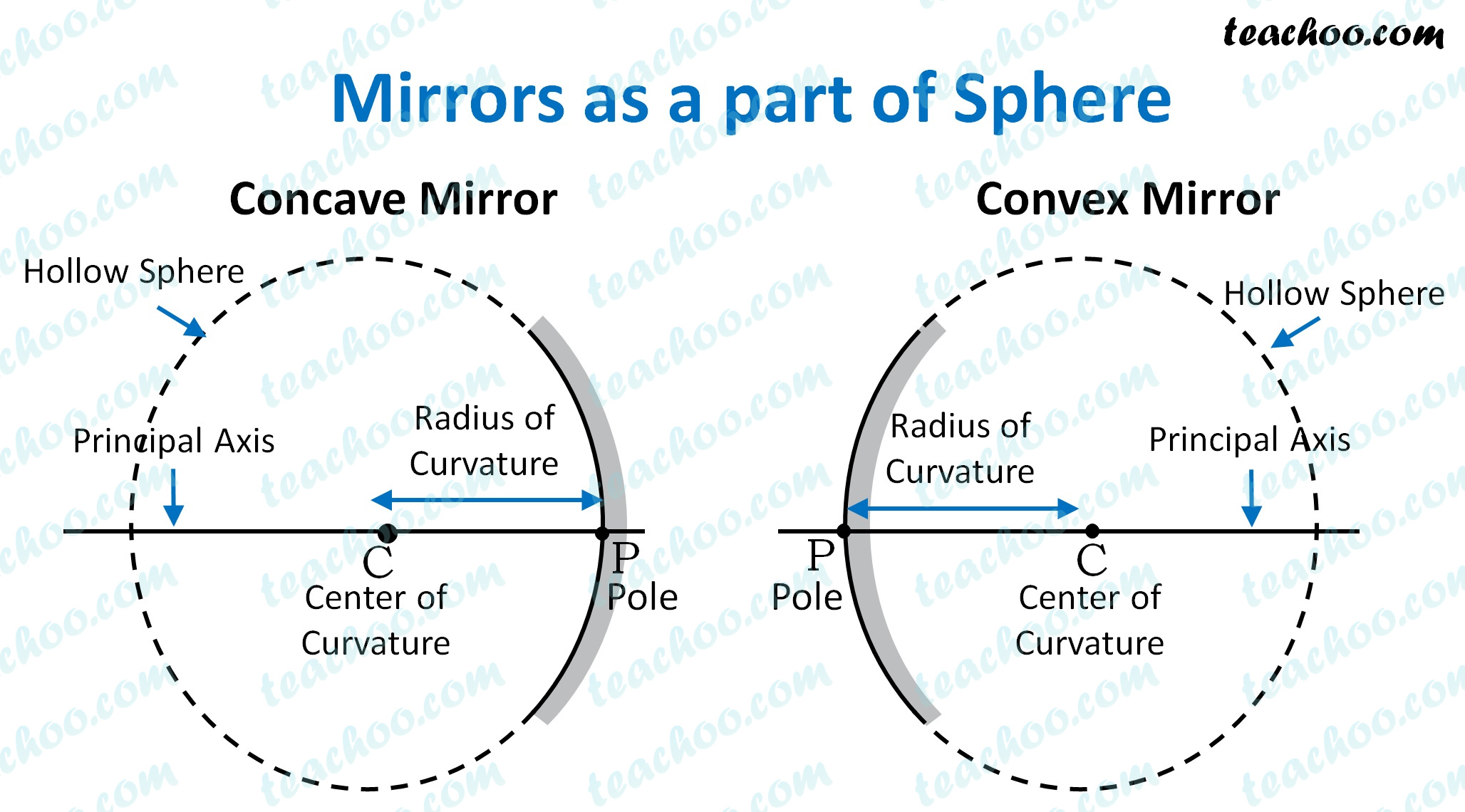Assertion Reasoning Questions (MCQ)

Class 10
Chapter 10 Class 10 - Light - Reflection and Refraction

## Reason (R): The focal length of a convex mirror of  radius R is equal to, f =  R/2  .

Here,

• Focal length of the spherical mirror  does not depend on the medium in which it is  placed.
Thus, focal length of convex mirror will remain the same.

• For spherical mirrors, Focal length = (Radius of Curvature/2)Thus,

• Assertion is false
• Reasoning is true

So, the correct answer is (d)

Get live Maths 1-on-1 Classs - Class 6 to 12